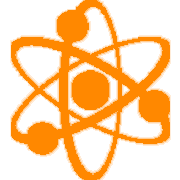# BPS District Science Standards Book

## HS Science Concepts

### [PS] Physical ScienceHigh School Science
"I can ... statements"In Physical Sciences, the high school level focus on students developing understanding of several scientific practices. These include developing and using models, planning and conducting investigations, analyzing and interpreting data, using mathematical and computational thinking, and constructing explanations; and to use these practices to demonstrate understanding of the core ideas. Students are also expected to demonstrate understanding of several of engineering practices including design and evaluation.

### SCI-HS.PS1 DCI:

• SCI-HS.PS1.01  Use the periodic table as a model to predict the relative properties of elements based on the patterns of electrons in the outermost energy level of atoms.
• SCI-HS.PS1.02  Construct an explanation for the outcome of a simple chemical reaction based on the outermost electron states of atoms, trends in the periodic table, and knowledge of the patterns of chemical properties.
• SCI-HS.PS1.03  Plan and conduct an investigation to gather evidence to compare the structure of substances at the macro scale to infer the strength of electrical forces between particles.
• SCI-HS.PS1.04  Develop a model to illustrate that the release or absorption of energy from a chemical reaction system depends upon the changes in total bond energy.
• SCI-HS.PS1.05  Apply scientific principles and evidence to provide an explanation about the effects of changing the temperature or concentration of the reacting particles on the rate at which a reaction occurs.
• SCI-HS.PS1.06  Refine the design of a chemical system by specifying a change in conditions that would produce increased amounts of products at equilibrium.
• SCI-HS.PS1.07  Use mathematical representations to support the claim that atoms, and therefore mass, are conserved during a chemical reaction.
• SCI-HS.PS1.08  Develop models to illustrate the changes in the composition of the nucleus of the atom and the energy released during the processes of fission, fusion, and radioactive decay.

### SCI-HS.PS2 DCI:

• SCI-HS.PS2.01  Analyze data to support the claim that Newton’s second law of motion describes the mathematical relationship among the net force on a macroscopic object, its mass, and its acceleration.
• SCI-HS.PS2.02  Use mathematical representations to support the claim that the total momentum of a system of objects is conserved when there is no net force on the system.
• SCI-HS.PS2.03  Apply scientific principles, such as Newton's 1st & 3rd Laws, and engineering ideas to design, evaluate, and refine a device that minimizes the force on a macroscopic object during a collision.
• SCI-HS.PS2.04  Use mathematical representations of Newton’s Law of Gravitation and Coulomb’s Law to describe and predict the gravitational and electrostatic forces between objects.
• SCI-HS.PS2.05  Plan and conduct an investigation to provide evidence that an electric current can produce a magnetic field and that a changing magnetic field can produce an electric current.
• SCI-HS.PS2.06  Communicate scientific and technical information about why the molecular-level structure is important in the functioning of designed materials.

### SCI-HS.PS3 DCI:

• SCI-HS.PS3.01  Create a mathematical model to calculate the change in the energy of one component in a system when the change in energy of the other component(s) and energy flows in and out of the system are known.
• SCI-HS.PS3.02  Develop and use models to illustrate that energy is associated with motion and relative position of particles (objects).
• SCI-HS.PS3.03  Design, build, and refine a device that works within given constraints to convert one form of energy into another form of energy.
• SCI-HS.PS3.04  Plan and conduct an investigation to provide evidence that the transfer of thermal energy when two components of different temperature are combined within a closed system results in a more uniform energy distribution among the components in the system (second law of thermodynamics).
• SCI-HS.PS3.05  Develop and use a model of two objects interacting through electric or magnetic fields to illustrate the forces between objects and the changes in energy of the objects due to the interaction.

### SCI-HS.PS4 DCI:

• SCI-HS.PS4.01  Use mathematical representations to support a claim regarding relationships among the frequency, wavelength, and speed of waves traveling in various media.
• SCI-HS.PS4.02  Evaluate the claims, evidence, and reasoning behind the idea that electromagnetic radiation can be described either by a wave model or a particle model, and that for some situations one model is more useful than the other.
• SCI-HS.PS4.03  Evaluate the validity and reliability of claims in published materials of the effects that different frequencies of electromagnetic radiation have when absorbed by matter.
• SCI-HS.PS4.04  Evaluate the validity and reliability of claims in published materials of the effects that different frequencies of electromagnetic radiation have when absorbed by matter.